# 7. Mixture and Alligation, Quantitative Aptitude, Civil Service Examination, RPSC Notes | Study RAS RPSC Prelims Preparation - Notes, Study Material & Tests - UPSC

## UPSC: 7. Mixture and Alligation, Quantitative Aptitude, Civil Service Examination, RPSC Notes | Study RAS RPSC Prelims Preparation - Notes, Study Material & Tests - UPSC

The document 7. Mixture and Alligation, Quantitative Aptitude, Civil Service Examination, RPSC Notes | Study RAS RPSC Prelims Preparation - Notes, Study Material & Tests - UPSC is a part of the UPSC Course RAS RPSC Prelims Preparation - Notes, Study Material & Tests.
All you need of UPSC at this link: UPSC

1. Alligation

It is the rule which enables us to find the ratio in which two or more ingredients at the given price must be mixed to produce a mixture of a specified price.

2. Mean Price

Mean price is the cost price of a unit quantity of the mixture

3. Suppose a container contains x of liquid from which y units are taken out and replaced by water. After n operations, the quantity of pure liquid = [x(1−y/x)n] units.

4. Rule of Alligation

If two ingredients are mixed, then

(Quantity of cheaper/Quantity of dearer)=(C.P. of dearer - Mean Price)/(Mean price - C.P. of cheaper)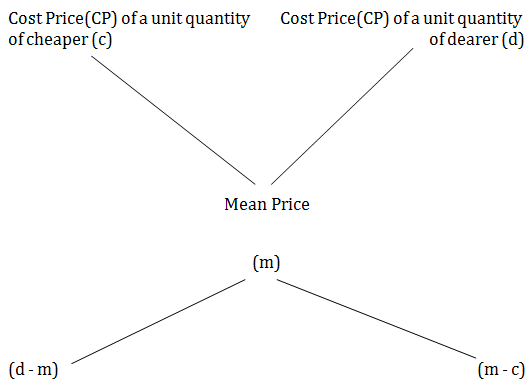5. => (Cheaper quantity) : (Dearer quantity) = (d - m) : (m - c)

Solved Examples

 1. A container contains 40 litres of milk. From this container 4 litres of milk was taken out and replaced by water. This process was repeated further two times. How much milk is now contained by the container? A. 26 litres B. 29.16 litres C. 28 litres D. 28.2 litres

Explanation :

Assume that a container contains x of liquid from which y units are taken out and replaced

=[x(1−y/x)n]

Hence milk now contained by the container = 40(1−4/40)3=40(1−1/10)3

40×9/10×9/10×9/10=(4×9×9×9)/100=29.16

 2. A jar full of whiskey contains 40% alcohol. A part of this whisky is replaced by another containing 19% alcohols and now the percentage of alcohol was found to be 26%. The quantity of whisky replaced is A. 43 B. 34 C. 32 D. 23

Explanation :

Concentration of alcohol in 1st Jar = 40%

Concentration of alcohol in 2nd Jar = 19%

After the mixing, Concentration of alcohol in the mixture = 26%

By the rule of alligation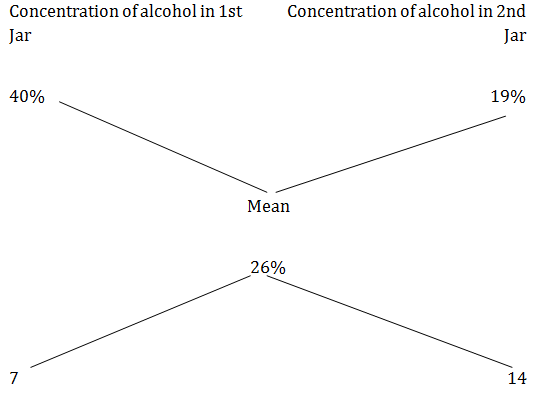Hence ratio of 1st and 2nd quantities = 7 : 14 = 1 : 2

 3. In what ratio should rice at Rs.9.30 per Kg be mixed with rice at Rs. 10.80 per Kg so that the mixture be worth Rs.10 per Kg ? A. 7 : 8 B. 8 : 7 C. 6 : 7 D. 7 ; 6

Explanation :

By the rule of alligation, we have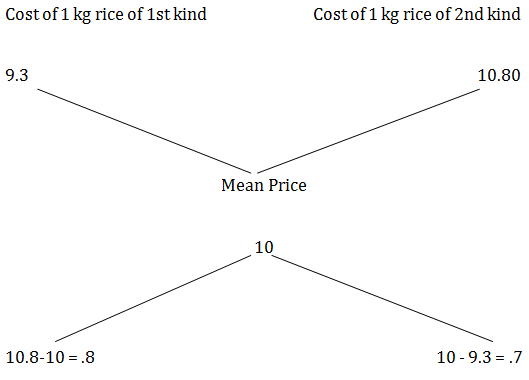Required ratio = .8 : .7 = 8 : 7.

 4. In what ratio must water be mixed with milk costing Rs.12 per litre in order to get a mixture worth of Rs.8 per litre? A. 1 : 3 B. 2 : 2 C. 1 : 2 D. 3 : 1

Explanation:

By the rule of alligation, we have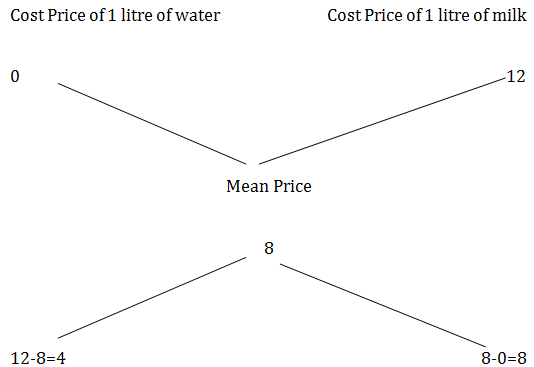Required Ratio = 4 : 8 = 1 : 2

 5. In 1 kg mixture of iron and manganese 20% of manganese. How much iron should be added so that the proportion of manganese becomes 10% A. 1.5 Kg B. 2 Kg C. .5 Kg D. 1 Kg

Explanation :

By the rule of alligation, we have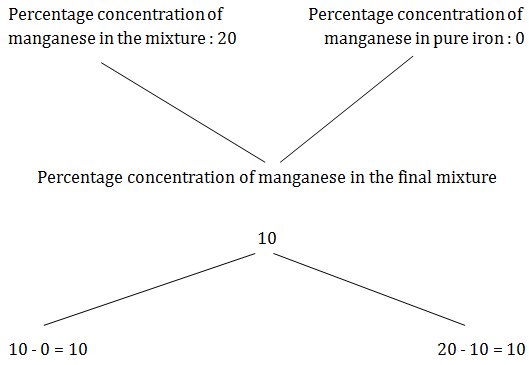=> Quantity of the mixture : Quantity of iron = 10 : 10 = 1 : 1

Given that Quantity of the mixture = 1 Kg

Hence Quantity of iron to be added = 1 Kg

 6. A trader has 1600Kg of sugar. He sells a part at 8% profit and the rest at 12% profit. If he gains 11% on the whole , find the quantity sold at 12%. A. 1200 Kg B. 1400 Kg C. 1600 Kg D. 800 Kg

Explanation :

By the rule of alligation, we have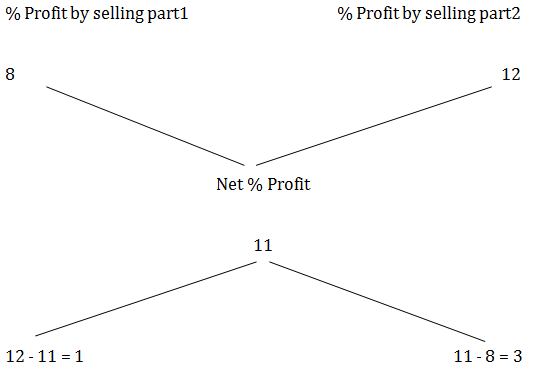=>Quantity of part1 : Quantity of part2 = 1 : 3

Given that total quantity = 1600 Kg

Hence quantity of part2 (quantity sold at 12%) = 1600×3/4=1200

 7. How many litres of water must be added to 16 liters of milk and water contains 10% water to make it 20% water in it A. 4 litre B. 2 litre C. 1 litre D. 3 litre

Explanation :

By the rule of alligation, we have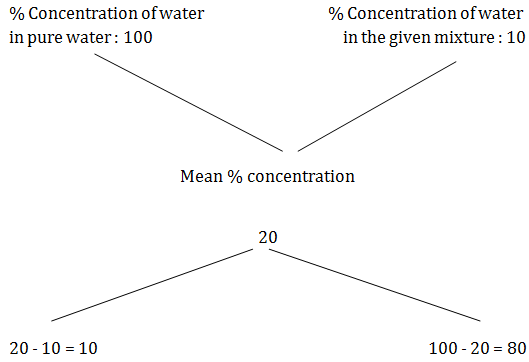=> Quantity of water : Quantity of the mixture = 10 : 80 = 1 : 8

Here Quantity of the mixture = 16 litres

=> Quantity of water : 16 = 1 : 8

Quantity of water = 16×1/8=2 litre

 8. A merchant has 1000 kg of sugar part of which he sells at 8% profit and the rest at 18% profit. He gains 14% on the whole. The Quantity sold at 18% profit is A. 300 B. 400 C. 600 D. 500

Explanation :

By the rule of alligation,we have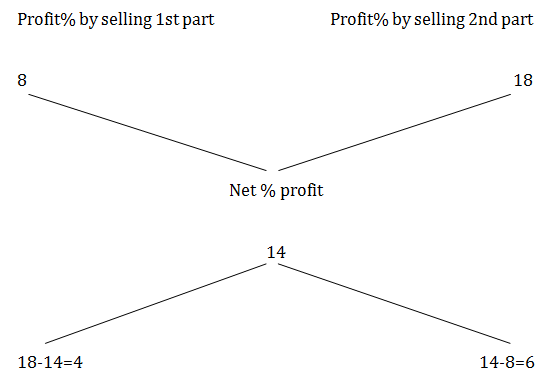=> Quantity of part1 : Quantity of part2 = 4 : 6 = 2 : 3

Total quantity is given as 1000Kg

So Quantity of part2 (Quantity sold at 18% profit) = 1000×3/5=600Kg

 9. Tea worth Rs. 126 per kg and Rs. 135 per kg are mixed with a third variety of tea in the ratio 1 : 1 : 2. If the mixture is worth Rs. 153 per kg, what is the price of the third variety per kg ? A. Rs.182.50 B. Rs.170.5 C. Rs.175.50 D. Rs.180

Explanation :

Tea worth Rs. 126 per kg and Rs. 135 per kg are mixed in the ratio 1 : 1

So their average price = (126+135)2=130.5

Hence let's consider that the mixture is formed by mixing two varieties of tea.

one at Rs. 130.50 per kg and the other at Rs. x per kg in the ratio 2 : 2, i.e.,

1 : 1. Now let's find out x.

By the rule of alligation, we can write as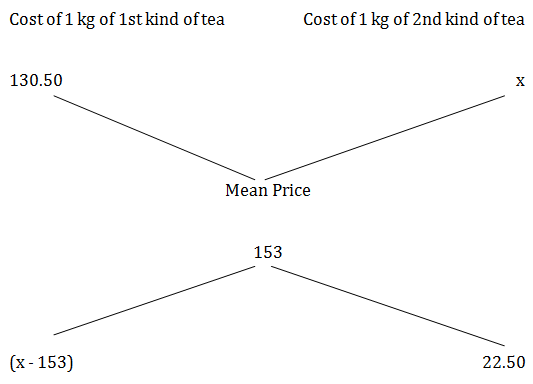(x - 153) : 22.5 = 1 : 1

=>x - 153 = 22.50

=> x = 153 + 22.5 = 175.5

 10. A milk vendor has 2 cans of milk. The first contains 25% water and the rest milk. The second contains 50% water. How much milk should he mix from each of the containers so as to get 12 litres of milk such that the ratio of water to milk is 3 : 5? A. 5litres, 7 litres B. 7litres, 4 litres C. 6litres, 6 litres D. 4litres, 8 litres

Explanation :

Let cost of 1 litre milk be Rs.1

Milk in 1 litre mix in 1st can = 3/4 litre

Cost Price(CP) of 1 litre mix in 1st can = Rs.3/4

Milk in 1 litre mix in 2nd can = 1/2 litre

Cost Price(CP) of 1 litre mix in 2nd can = Rs.1/2

Milk in 1 litre of the final mix=5/8

Cost Price(CP) of 1 litre final mix = Rs.5/8

=> Mean price = 5/8

By the rule of alligation, we can write as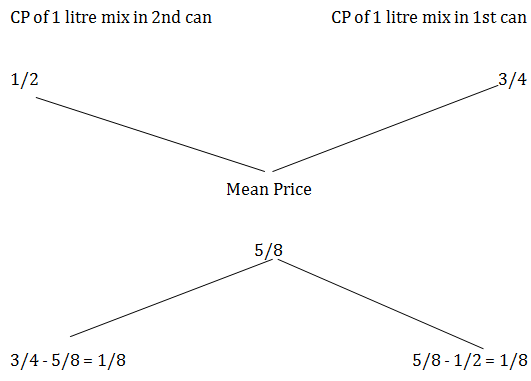=> mix in 2nd can :mix in 1st can = 1/8 : 1/8 = 1:1

ie, from each can, 12×12=6 litre should be taken

The document 7. Mixture and Alligation, Quantitative Aptitude, Civil Service Examination, RPSC Notes | Study RAS RPSC Prelims Preparation - Notes, Study Material & Tests - UPSC is a part of the UPSC Course RAS RPSC Prelims Preparation - Notes, Study Material & Tests.
All you need of UPSC at this link: UPSCUse Code STAYHOME200 and get INR 200 additional OFF

## RAS RPSC Prelims Preparation - Notes, Study Material & Tests

109 docs|21 tests

### How to Prepare for UPSC

Read our guide to prepare for UPSC which is created by Toppers & the best Teachers

Track your progress, build streaks, highlight & save important lessons and more!

,

,

,

,

,

,

,

,

,

,

,

,

,

,

,

,

,

,

,

,

,

,

,

,

,

,

,

,

,

,

,

,

,

;